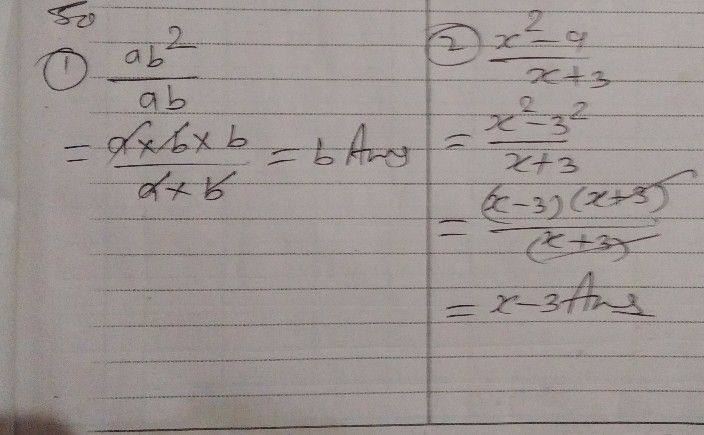Symbol
Problem$4y.+10$ $'$ $\dfrac {t>\right)\left(} {,s\right)\left(.},\dfrac {>} {2\right)}=$ $-2$ Learning Task 2. Simplity the following rational expressions. $10$ it in your notebook. 1. $\dfrac {ab^{2}} {ab}$ $2.\dfrac {x^{2}-9} {x+3}$ $3$ $\dfrac {4x^{2a.+}} {\bar{x} -3}^{12}$ $4.\dfrac {x^{2}+3} {x^{2}-}^{x-4}$ $1$ E Learning Task $3$ Encircle Rational Algebraic Expression. $D0$ this $111$ your notebook.
7th-9th grade
Algebra
Search count: 106
SolutionQanda teacher - Nirmalya